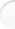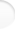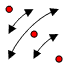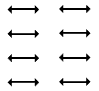Structure of Magic and Semi-Magic Squares, Methods and Tools for Enumeration by Francis Gaspalou1. Definition of a Transformation

The 8 transformations of the square (identity, vertical symmetry, horizontal symmetry, symmetry about the first diagonal, symmetry about the second diagonal, rotation 90° clockwise, rotation 90° anticlockwise, symmetry about the centre) are well known. But other transformations exist.

A transformation between 2 (magic or semi-magic) squares of the same order n is an application which makes a correspondence between each cell of the first square and one and only one other cell of the second square. It is an operator on the n² cells or a permutation of these n² cells.

Two squares of the same order are sufficient to define a transformation. According to the position of the number 1 in the first and in the second squares, a law (or an application) is defined between the two cells occupied by this number 1; idem for the number 2, ...until the number n² (I consider here normal magic or semi-magic squares, i.e. magic or semi-magic squares made with the numbers 1 to n²).

A transformation has a matrix of definition and a graph of definition (it is an oriented graph).
For example, the transformation "symmetry about the first diagonal" for squares of order 3 transforms an initial square:

 A1 A2 A3 B1 B2 B3 C1 C2 C3

into the transformed square:

 A1 B1 C1 A2 B2 C2 A3 B3 C3

To define a transformation, it is useful to write only the transformed square, the initial square is not written. But the matrix for the transformed square is not only the image of a square, it is also the image of an operator on the position of the cells (matrix of definition of this transformation).
The oriented graph (or diagram) is more significant:If we call G this transformation, we can write for example:

 2 7 6 9 5 1 4 3 8*
G  =
 2 9 4 7 5 3 6 1 8

It should be possible to define a transformation by a permutation under the n² cells - permutation on the position of cells -; for example for the symmetry about the first diagonal:
 ↓ A1 A2 A3 B1 B2 B3 C1 C2 C3 A1 B1 C1 A2 B2 C2 A3 B3 C3
but such a notation is not usual .

 2. Properties of Transformations Top of the pageA transformation is defined a priori (e.g.: vertical symmetry). But the interesting transformations are those that keep the magic properties for the transformed square. Therefore, a transformation has a space of definition on a given field: it is the set of squares that satisfy the magic conditions for the transformed square. By field, I mean for example the set of magic squares of order 4.
 E.g.: the transformation which is a permutation of the two first columns and of the two last columns, on the 7 040 magic squares of order 4:

 A2 A1 A4 A3 B2 B1 B4 B3 C2 C1 C4 C3 D2 D1 D4 D3
orThe magic conditions are A2+B1+C4+D3=34 and A3+B4+C1+D2=34. You find that the sum of these two conditions is an identity (it is true for all the magic squares) and finally there is only one condition.
With a computer, you find that there are 3 456 solutions among the 7 040: the 3 456 semi-pandiagonal squares. It is the space of definition of the above mentioned transformation.

The space of definition is transformed into a transformed space, or image space.

A transformation is bijective (each element has only one image and reciprocally). The definition space and the image space are equipotent (they have the same number of elements) but they are also isomorphic (they have the same form or structure).

Any transformation has its inverse transformation.

Transformations can be composed: the product of two transformations is the classical product of two matrix.

A transformation has a period p (or an order p), that is to say you find the transformation identity when you apply p times the transformation on itself. The graph is very useful to look at a glance this order p.

 3. Definition of Sets of Squares Top of the pageI define sets of squares as sets having in common several relations of definition.

Examples:
• the magic squares of order 4
• the pandiagonal squares of order 5
• the 3 456 semi-pandiagonal squares of order 4 (defined for example by the condition on the cells A1+A2+B1+B2=34)
• ...

The interesting sets are mainly the sets defined by conditions on the cells (and especially sum of n cells = magic constant).

For example, the space of definition of a transformation on a given field is very interesting (the conditions are then the magic conditions of the transformed square). Note that such a space can be defined by this transformation, but very often other transformations can also define the same space.

My definition of sets of squares is not mathematically very precise. In fact, you will see that these sets are defined by their properties (see next paragraph). I found first these properties and I tried after to give a definition of sets.

 4. Properties of the Sets of Squares Top of the page4.1 Internal transformations

For a given set of squares, I search first what I call the internal transformations.

A transformation is said to be internal to the set if for any square of the set, the transformed square belongs too to the set. The transformed space is then identical to the definition space.

I have the fundamental property:
the internal transformations of a set constitute a group called G.

For mathematicians, the transformations satisfy the 3 conditions of definition of a group: the transformations are associative, you have an identity element, each transformation has an inverse.

The group G is the group of highest order which operates on the set. All the groups which operate on the set are subgroups of G. In other words, the sets of squares are G-sets.

4.2 Examples

On the 7 040 squares of order 4, a group of order 32 operates. See Appendix 3, § 1.

See also this Appendix (and the page Main results) for general formulas giving the order of group G for semi-magic and for magic squares of order n. The last formula seems original.

4.3 Application

The notion of group G allows to reduce the enumeration of a given set of squares to the enumeration of the "elementary squares" or "essentially different squares". You have to search only these elementary squares.

For that, you search the internal transformations and then, the order of group G. Some transformations are obvious, the difficulty is to avoid to forget anyone. For that, you can use some tools.
For example, if the number of elementary squares is a prime, you are sure you have not any supplementary transformation.
You can also distribute the solutions according to the position occupied in the cells by a given number as number 1: if some cells have the same number of solutions, you can have ideas of transformations.
All these methods suppose you have computed the exact number of elementary squares. Otherwise, the problem is harder!

To determine the probable order of group G is a delicate but relatively easy task. But, to demonstrate that the group G has the supposed order is a very hard task, and to identify the group among all the groups of the given order is a very difficult task too.
For that, you can try different "abstract definitions" of groups, and then choose generators among the internal transformations.
I used this method to demonstrate that the group of order 32 for the magic squares of order 4 is G 32 n° 7 of BURNS.
I used also this method to demonstrate that the group of order 1 152, for the 3 456 semi-pandiagonal squares of order 4, is the only group of degree 8 of BURNS.
See Appendix 3, § 3.
Caution! You have to verify that the generators give the good group and not a subgroup: the number of generated elements should have the correct value.
Besides, the abstract definition of groups is not given in the literature for all the groups and catalogues of groups exist only for low orders (See for example the book of F.J. BUDDEN: The fascination of groups, page 299).
Note: the abstract definition of a group is not unique.

Therefore, sets of squares lead to search and to identify groups: it is really an Eldorado for amateurs! To find and to identify the group G of a given set is an intellectual challenge which needs to handle abstract definitions of groups. This kind of mathematics was very fashionable in the years 1890-1910, but nowadays it seems a little old-fashioned or out-of-date!

Note: for the group G, you have to determine all the transformations and not only the transpositions (transformations of rows, columns, and broken diagonals) as the literature often says.

For example, for the 28 800 pandiagonal squares of order 5, the literature seems to give only a group of order 800 and 36 essentially different squares. See for example the book of William H. BENSON and Oswald JACOBY: New recreations with magic squares. BENSON and JACOBY consider only transpositions; in fact the transformations between the 36 essentially squares - given by these authors - are good for all the squares and you see that all the 28 800 squares can be generated by only one square.

In the same idea of transpositions and transformations, the symmetrical pandiagonal squares of order 7 have a group G of order 96 (See Walter TRUMP's site and see also Appendix 6, § 2).
In fact, there are 48 transpositions and a supplementary transformation W of order 2 that I found. W is not a transposition.

Many questions are open, chiefly when the sets are not computed.
For example, what is the order of the group G for pandiagonal squares of order 7? You will see easily with the book of BENSON and JACOBY (page 140) that the order of the subgroup of transpositions is 2 352, but is it possible to find other transformations different from these transpositions? For pandiagonal squares of order 4 and 5, you have a lot of transformations different from transpositions, but for the moment I didn't find any equivalent transformation for order 7:

OrderNumber of
pandiagonal squares
Order of group GOrder of the subgroup
of transpositions
4384384128
528 80028 800800
60irrelevantirrelevant
7? (estimated by Walter TRUMP)2 352?2 352

I think the order of group G for 7x7 pandiagonal is 2 352 but I am not totally sure of it.

I made a program to calculate the transformations between the elementary cyclical pandiagonal squares of order 7 and I applied each transformation to an irregular pandiagonal square to see if the transformed square was pandiagonal: I didn't find any new transformation (whereas such a method gives good results for order 4 or 5: all the transformations between elementary cyclical pandiagonal squares belong to group G)! Therefore, for order 7, if a new transformation exists besides transpositions, it is not a transformation between the elementary cyclical pandiagonal squares.

4.4 Specific sets of squares

Generally, the order of group G is less than the dimension of the set of squares. But, for some specific sets, there is equality. These sets have a group structure.

This means that any transformation between two squares of the set can be applied to any other square of the set: the transformed square will belong to the set. This means too that all the set can be generated from only one square.

For example, it is true for 11 sets of 384 squares of order 4 (pandiagonal, symmetrical, ...- all with the same abstract definition - ), for the 28 800 pandiagonal squares of order 5, etc. These results seems original.

See Appendix 3, § 3 for the 3 456 semi-pandiagonal squares of order 4.

For pandiagonal squares of order 7, you have not a group (it is very easy to demonstrate: you take 2 pandiagonal squares and you see that the transformation between them is generally not an internal transformation).

4.5 External transformations

Some sets of squares may have, on a given field, one or several isomorphic sets, that is to say sets with the same structure. Then, it is possible to find a transformation called "external transformation" that makes a correspondence between each element of the first set and each element of the isomorphic set. This transformation is a bijection and the two sets are equipotent (they have the same number of elements).

The number of isomorphic sets is finite (when such sets exist).

To find these isomorphic sets is a difficult task. The problem is to identify these external transformations. You can apply the group G of the field and search the transformations which are not internal transformations in the given set. But you can have external transformations which are not transformations of the group G of the field: the set may belong to an intermediate set - defined on the field - and you have then to apply the group G of this intermediate set. Very often, such an intermediate set is not unique.

At the difference of internal transformations that constitute a group, the external transformations generally don't constitute a group on the given field. In fact, the group exists but on a larger field: you have to add other squares and these additional squares are not necessarily magic.

The isomorphic squares are not necessarily disjointed, but they can be so.

The set with its isomorphic sets will be called the complete set.

For example, the 384 symmetrical squares of order 4 have 10 isomorphic sets on the field of the 7 040 magic squares of order 4: 8 constitute - with these 384 symmetrical - the set of 3 456 semi-pandiagonal squares (on which operates a group of order 1 152), but there are 2 supplementary sets. Finally, you have 11 disjointed sets of 384 squares, and a complete set of 4 224 squares.

 5. Structure of Magic and Semi-Magic Squares Top of the page5.1 Structure of magic squares of order 4

It is usual to distribute the 7 040 squares of order 4 in types (graph made with pairs of numbers, the sum of each pair being n² + 1=17).

You obtain:
• 25 different types (symmetrical, pandiagonal,...)
• 12 types if you consider as a unique type the types given by the octic group of the square (these 12 types are classical and known as DUDENEY's types)
• 10 types if you consider as a unique type the types given by the group G of 32
• 4 basic types if you consider as a unique type the types given by bijection (each bijection being specific of each type).

Therefore, the 7 040 squares can be finally decomposed in only 4 basic types n° 1 to 4 (this result seems original):

Basic typesDUDENEY's
types
Number of
squares for
each type
Number of
isomorphic
sets (with
basic set
included)
Total
number of
squares
with
isomorphic
sets
Number K
of magic
series for
each type
Number N
of
parameters
for each
type
n° 1I,II,III,IV,V38472 688524
n° 2VI1 21622 432345
n° 3VII,VIII,IX,X22481 792444
n° 4XI,XII168   128563
4 basic types25 types7 040

A magic series is a sum of 4 numbers a+b+c+d=34 (34 is the magic constant). They are 86 different magic series for order 4.

The total set of 7 040 squares is a set with N=7 parameters and with K=14 magic series:
• 4 rows + 4 columns + 2 diagonals,
• A1+A4+D1+D4=34 (4 corners),
• B2+B3+C2+C3=34 (centre),
• A2+A3+D2+D3=34 ,
• B1+B4+C1+C4=34.

In the classification in 4 basic types, note you don't take by bijection all the isomorphic sets in each type (the squares of type n° 1 are semi-pandiagonal, but there are also 384 semi-pandiagonal squares in the type n° 2). Note also that if the 4 basic types are disjointed, the associated complete sets are not.

The 4 basic types are not totally independent: each set is isomorphic to a part of another larger set and it is get when you give supplementary conditions to this larger set, conditions sum of n cells = 34. All the 4 sets come by filiation from a set of 27 648 semi-magic squares of DUDENEY's type VI. There are 2 filiations (you have to add also a set of 3 072 semi-magic squares of DUDENEY's type IX):For this graph of filiation, see some explanations in Appendix 3, § 4.

Other classifications than that in types are possible. In the page The intermediate square method, § 5, I suggest another classification. This classification in disjointed sets of intermediate squares is also a classification in disjointed complete sets.

5.2 Structure of semi-magic squares of order 4

I applied the same method to the classification in types of semi-magic squares of order 4.

I obtained 3 495 different types (equivalent to the 25 types for magic squares of order 4) or 11 basic types (with their isomorphic sets) and a graph of filiation (but much more complicated!).

5.3 Conjecture: structure of magic squares and semi-magic squares of order n

The classification in basic types is only possible for magic and semi-magic squares of order 4. For higher orders, the number of types is very important and the calculus inextricable.

I suppose - but it is only a conjecture - that magic squares and semi-magic squares of order n can be decomposed in basic sets. For each basic set, it is necessary to associate some isomorphic sets (but not all the isomorphic sets).

Then, the basic sets constitute an alphabet which allows to describe all the set of magic or semi-magic squares.

The basic sets are not totally independent: each set is isomorphic to a part of another larger set and it is get when you give supplementary conditions to this larger set, conditions sum of n cells = magic constant. All the basic sets come by filiation from only one set and you can draw a graph of filiation.

5.4 Mathematical structure of magic squares and semi-magic squares of order n

This paragraph is rather for mathematicians.

In fact, magic squares of order n and semi-magic squares of order n are vector spaces.

I think the best books of reference in this domain are (sorry, but they are in French and their level is very high!):
• B. BELOUZE, M. GLAYMANN, P.-J. HAUG et J.-C. HERZ, Les carrés magiques, édition A.P.M.E.P. 1975
• J. M. GROIZARD, Algèbre des carrés magiques, édition A.P.M.E.P. 1984

The literature gives the dimension of these vector spaces: (n-1)2 - 1 for magic squares of order n [e.g.: for n=4, this dimension is 8] and (n-1)2 + 1 for semi-magic squares of order n.
You can compare this dimension to the number of parameters necessary to compute the set. This number is classically 7 for magic squares of order 4, (n-1)2 - 2 for magic squares of order n and (n-1)2 for semi-magic squares of order n, but with numbers from 1 to n² [you need (n-1) parameters in the first row, (n-1) other parameters in the second row, ...]. If you don't want that the numbers should be between 1 and n², you find the same numbers for the dimension of the vector space and for the number of parameters.

The problem is to find a basis for each of these vector spaces.
With a basis, each magic square can be written as a linear combination. For example, each magic square of order 4 has 8 coordinates in the vector space of dimension 8; these 8 coordinates are the 8 parameters that define this magic square; there is only one way to define this magic square in a given basis.
GROIZARD gives an example of basis for magic squares of order 4, basis made from matrix 4x4 with only 0, 1 or -1 inside:

 0 1 -1 0 -1 0 0 1 1 0 0 -1 0 -1 1 0

A0
 1 1 1 1 1 1 1 1 1 1 1 1 1 1 1 1

B0
 1 0 0 -1 0 -1 1 0 0 1 -1 0 -1 0 0 1

B1
 0 1 -1 0 1 0 0 -1 -1 0 0 1 0 -1 1 0

B2

 0 1 0 -1 -1 0 1 0 0 -1 0 1 1 0 -1 0

D1
 0 0 1 -1 0 0 -1 1 -1 1 0 0 1 -1 0 0

D2
 -1 0 1 0 0 1 0 -1 1 0 -1 0 0 -1 0 1

C1
 -1 1 0 0 1 -1 0 0 0 0 1 -1 0 0 -1 1

C2

Note: I changed A of GROIZARD in A0.

GROIZARD explains that a group of order 16 operates on this vector space (in fact, there is a larger group of order 32). GROIZARD explains also that a group of order 64 operates on symmetrical squares (you can do better: in fact, it is a group of order 384). You have in this book the beginning of my theory of groups which operate on vector spaces.

I can make the link between the 8 GROIZARD's coordinates a0, b0, b1, b2, d1, d2, c1, c2 of a magic square of order 4 and the 7 parameters (for example A, B, C, D, E, F, G) used in my computing program:

 A F 34-A-C-F C G D E 34-D-E-G B+C-G A+B-E 34-A-B-D D+E+G-B-C 34-A-B-C 34-A-B-D+E-F 2*A+B+C+D-E+F-34 B

As any magic square of order 4 can be written as
a0A0+b0B0+b1B1+b2B2+d1D1+d2D2+c1C1+c2C2
I can also write 7 relations:
A = b0 + b1 - c1 - c2,
B = b0 + b1 + c1 + c2,
...
Then I find:
a0=(A+B+C-E+F-G-17)/2   b0=17/2         b1=(A+B-17)/2         b2=(A+D+F+G-34)/2
d1=(17-A-B-C+E)/2             d2=(17-C-E)/2          c1=(B+D-17)/2          c2=(17-A-D)/2

Therefore, from the values of the 7 parameters of each 220 elementary squares of order 4 given by my computer, I can have the values a0, b0, b1, b2, d1, d2, c1, c2 for each elementary square:

Number2*a02*b02*b12*b22*d12*d22*c12*c2
1017-12-41212
2-417-1201212
...........................
220........................

and then also for the 7 040 magic squares which can now be written as a linear combination of these new parameters : you see the importance of the choice of the parameters!

These data are very interesting:
a0=0 gives 1 152 solutions: 384 symmetrical + the two supplementary sets of 384 squares of DUDENEY's
type VI isomorphic to semi-pandiagonal squares (but yet not semi-pandiagonal)
b1=0 gives 384 solutions: the 384 symmetrical
b2=0 gives 3 456 solutions: the 3 456 semi-pandiagonal solutions
d1=0 gives 384 solutions: the 384 pandiagonal
d2=0 gives 384 solutions: the 384 squares of DUDENEY's type II
c1=0 gives 384 solutions: the 384 pandiagonal
c2=0 gives 384 solutions: the 384 squares of DUDENEY's type II

The 384 symmetrical squares are generated by {C1, C2, D1, D2}, that is to say a0=b1=b2=0.

We see we have here the equations of the main sets among magic squares.

BELOUZE, GLAYMANN, HAUG and HERZ demonstrate that a basis for semi-magic squares of order n can be obtained with permutation matrix (matrix with 0 and 1 only, and with one element 1 in each row and column). They show an example of decomposition of a given square. They mention also a general method of decomposition (Hungarian algorithm).

I think the choice of parameters (in a computing program) as coordinates in a basis of the vector space - or the link between parameters and coordinates in the vector space - is a very interesting problem to explore. I don't know any publication on this subject.